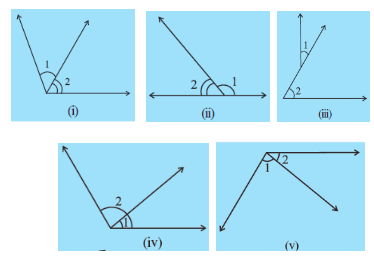Q

# Are the angles marked 1 and 2 adjacent? (Fig ). If they are not adjacent, say, ‘why’.

1.  Are the angles marked 1 and 2 adjacent? (Fig). If they are not adjacent, say, ‘why’.Views

The condition for being adjacent angles are:-

(a)  they have a common vertex

(b)  they have common arm

Hence in the given figures:-

(i)  These angles are adjacent angles as they agree above conditions.

(ii) The angles are adjacent angles.

(iii) These angles are not adjacent as their vertices are different.